# 多因素驱动的配电变压器时变故障失效模型及应用Time-Varying Failure Model of Multi-Factor Driven Distribution Transformer and Its Application

DOI: 10.12677/SG.2019.96028, PDF, HTML, XML, 下载: 290  浏览: 746

Abstract: Most of distribution system reliability evaluation is based on the average failure rate of the long-term historical data of the equipment, which cannot respond to the dynamic changes of the operation status and the operation environment. When synthetically considering the failure model of transformer caused by thermal aging, discharge, damp and other factors, the paper presents a failure model of environmental temperature and load characteristics, with the failure model based on health status. Therefore, we can gain the short-term reliability model of transformer driven by multi factors. With the reliability evaluation index constructed of distribution network, the short-term reliability evaluation of distribution network is realized by using the forward fault diffusion method based on the short-term reliability model of transformer. Finally, the validity of the short-term reliability model of the transformer is verified by a test system. The result can realize the fault early warning, and provide the basis for the rapid troubleshooting of the distribution network.

1. 引言

2. 多因素驱动的变压器故障率模型

2.1. 负荷特性与环境温度相依的老化失效模型

${\theta }_{\text{oil}}={\left(\frac{{k}^{2}R+1}{R+1}\right)}^{\frac{1}{1+n}}{\mu }_{\text{pu}}^{\frac{n}{n+1}}\Delta {\theta }_{\text{oil,R}}+{\theta }_{\text{a}}$ (1)

${\mu }_{\text{pu}}=\frac{\mu }{{\mu }_{\text{R}}}=\mathrm{exp}\left(\frac{2797.3}{{\theta }_{\text{oil}}+273}-\frac{2797.3}{{\theta }_{\text{oil,R}}+273}\right)$ (2)

${\theta }_{\text{hst}}={k}^{\frac{2m}{n+1}}{\mu }_{\text{pu}}^{\frac{n}{n\text{+1}}}\Delta {\theta }_{\text{hst,R}}\text{+}{\theta }_{\text{oil}}$ (3)

$V=\mathrm{exp}\left(\frac{B}{110+273}-\frac{B}{{\theta }_{\text{hst}}+273}\right)$ (4)

$T=N/V$ (5)

$T=N\mathrm{exp}\left(\frac{15000}{{\theta }_{\text{hst}}+273}-\frac{15000}{110+273}\right)$ (6)

$\lambda \left(k,{\theta }_{\text{a}}\right)=\frac{1}{T}N\mathrm{exp}\left(\frac{15000}{110+273}-\frac{15000}{{\theta }_{\text{hst}}+273}\right)$ (7)

2.2. 健康状态相依的潜伏性失效模型

$\lambda \left(\text{HI}\right)=k{\text{e}}^{-c\text{HI}}$ (8)

2.3. 健康状态相依的潜伏性失效模型

${\lambda }_{\text{t}}=\lambda \left(\text{HI}\right)+\lambda \left(k,{\theta }_{\text{a}}\right)$ (9)

${t}_{\text{t}}=\frac{\lambda \left(k,{\theta }_{a}\right){t}_{1}+\lambda \left(\text{HI}\right){t}_{2}+\lambda \left(k,{\theta }_{a}\right){t}_{1}\lambda \left(\text{HI}\right){t}_{2}}{{\lambda }_{\text{t}}}$ (10)

3. 输电线路安全裕度的表征配电网的短期风险评估

3.1. 用户失负荷时间期望(LLTE)

$\text{LLTE}=\frac{\underset{i\in \text{NS}}{\sum }\left({U}_{\text{LP,}i}{N}_{i}\right)}{\underset{i\in \text{NS}}{\sum }{N}_{i}}$ (11)

3.2. 用户失负荷概率期望(LLPE)

$\text{LLPE}=\frac{\underset{i\in \text{NS}}{\sum }\left({\lambda }_{\text{LP,}i}{N}_{i}\right)}{\underset{i\in \text{NS}}{\sum }{N}_{i}}$ (12)

3.3. 用户失负荷平均持续时间(LLDE)

$\text{LLDE}=\frac{\underset{i\in \text{NS}}{\sum }\left({U}_{\text{LP,}i}{N}_{i}\right)}{\underset{i\in \text{NS}}{\sum }\left({\lambda }_{\text{LP,}i}{N}_{i}\right)}$ (13)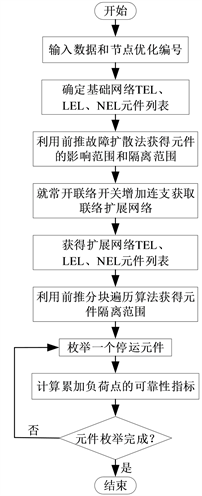Figure 1. Reliability assessment algorithm flow

4. 导线动态载流能力评估与运行温度预警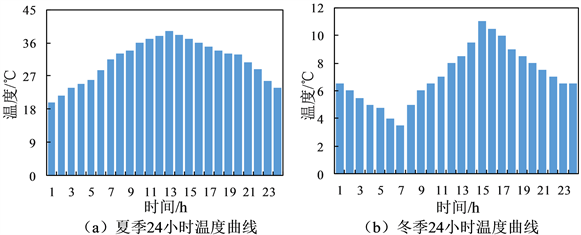Figure 2. 24-hour ambient temperature curve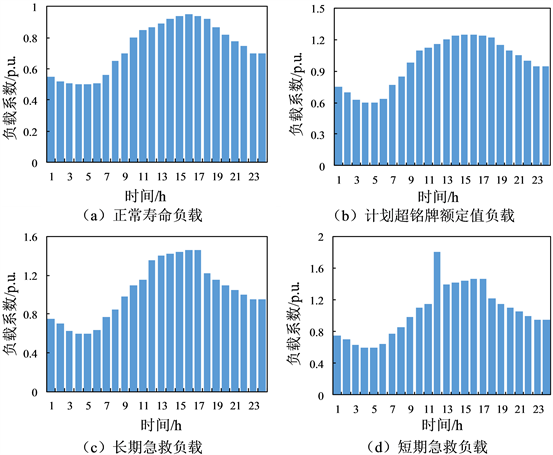Table 1. Transformer short-term reliability model parameters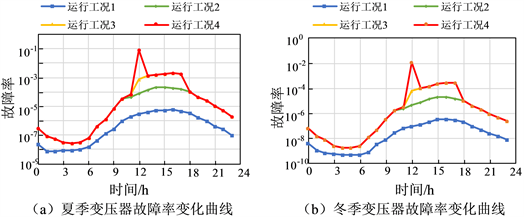Figure 4. Transformer real-time failure rate curve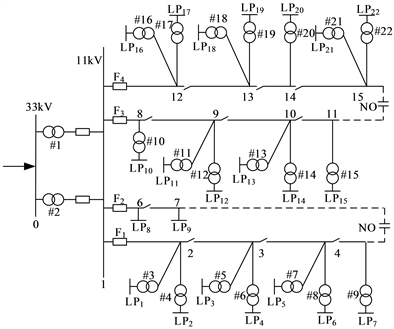Figure 5. RBTS-BUS2 distribution network

case1：假设RBTS-BUS2系统中的变压器承载正常周期性负载；

case2：假设RBTS-BUS2系统中#11变压器承载超铭牌过负荷运行，其余变压器承载正常周期性负载；

case3：假设RBTS-BUS2系统中的#11变压器承载长期急救负载运行，其余变压器承载正常周期性负载；

case4：RBTS-BUS2系统中#11变压器承载短期急救负载运行，其余变压器承载周期性负载。Table 2. Transformer short-term reliability model parametersFigure 6. LPE in RBTS-BUS2 system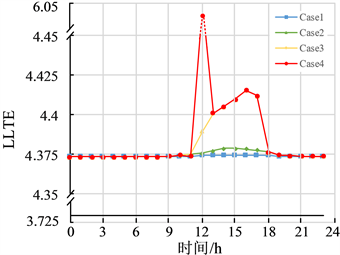Figure 7. LLTE in RBTS-BUS2 system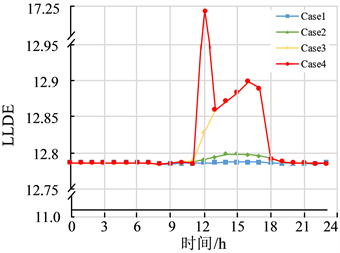Figure 8. LLDE in RBTS-BUS2 system

5. 结论

1) 变压器运行状态的动态变化会影响变压器的实时故障率，进而影响配电系统的可靠性评估指标，利用构建的可靠性指标有效地协助配电系统运行维护人员辨识配电网运行状态，为实现配电系统的故障预警提供底层基础。

2) 当变压器承载周期性负载以及计划超铭牌运行时，变压器的故障率主要由健康状态相依的潜伏性失效模型决定，当变压器承载长期急救性负载以及短期急救负载时，变压器的故障率主要由环境温度与负荷特性相依的实时故障率模型决定。

3) 基于变压器的短时可靠性模型的配电网可靠性评估，可获取配电网运行风险较大的时间点，可协助运行人员进行相应的系统调整，为实现配电网的故障预警以及故障快速排除提供基础。

  何英静, 赵伟, 朱艳伟, 等. 基于复杂网络理论的配电网中性点接地方式可靠性影响研究[J]. 电测与仪表, 2018, 55(22): 19-24.  安佳坤, 贺春光, 韩璟琳, 等. 基于配网特征指标的网格可靠性评估方法[J]. 电测与仪表, 1-11.  白恒远, 潘凯岩, 王森, 等. 基于拉格朗日函数的配电网评价指标权重研究[J]. 电测与仪表, 2019, 56(8): 1-8.  孙鹏, 陈绍辉, 张彩庆. 基于Marquardt法参数估计的变电设备寿命周期故障率评估[J]. 电力系统保护与控制, 2012, 40(1): 85-90.  何剑, 程林, 孙元章, 等. 条件相依的输变电设备短期可靠性模型[J]. 中国电机工程学报, 2009, 29(7): 39-46.  宁辽逸, 吴文传, 张伯明. 运行风险评估中的变压器时变停运模型(一)基于运行工况的变压器内部潜伏性故障的故障率估计方法[J]. 电力系统自动化, 2010, 34(15): 9-13  章禹, 何迪, 许奕斌, 等. 计及综合风险的油浸式变压器短期增容决策方法[J]. 电力系统自动化, 2017, 41(13): 86-91+118.  宁辽逸, 吴文传, 张伯明. 运行风险评估中的变压器时变停运模型(二)基于延迟半马尔可夫过程的变压器时变停运模型[J]. 电力系统自动化, 2010, 34(16): 24-28.  Susa, D., Lehtonen, M. and Nordman, H. (2005) Dynamic Thermal Modelling of Power Transformers. IEEE Transactions on Power Delivery, 20, 197-204. https://doi.org/10.1109/TPWRD.2004.835255  熊希聪, 王强钢, 周念成, 等. 基于谐波解析的电动汽车充电对配电变压器影响概率评估方法[J]. 电网技术, 2015, 39(8): 2283-2290.  潘乐真, 张焰, 俞国勤, 等. 状态检修决策中的电气设备故障率推算[J]. 电力自动化设备, 2010, 30(2): 91-94.  王慧芳, 杨荷娟, 何奔腾, 等. 输变电设备状态故障率模型改进分析[J]. 电力系统自动化, 2011, 35(16): 27-31.  彭卉, 张静, 吴延琳, 等. 基于可靠率指标的中压架空馈线分段优化[J]. 电力自动化设备, 2017, 37(5): 184-190.  谢开贵, 王小波. 计及开关故障的复杂配电系统可靠性评估[J]. 电网技术, 2008, 32(19): 16-21.  王秀丽, 罗沙, 谢绍宇, 等. 基于最小割集的含环网配电系统可靠性评估[J]. 电力系统保护与控制, 2011, 39(9): 52-58.  昝贵龙, 王主丁, 刘念祖, 等. 考虑容量及电压约束的高压配电网可靠性快速评估算法[J]. 电力系统自动化, 2017, 41(17): 121-127.  赵华, 王主丁. 复杂配网的前推分块遍历可靠性评估快速算法[J]. 电力系统及其自动化学报, 2016, 28(2): 85-92.  Swift, G., Molinski, T.S. and Lehn, W. (2002) A Fundamental Approach to Transformer Thermal Modeling. I. Theory and Equivalent Circuit. IEEE Transactions on Power Delivery, 16, 171-175. https://doi.org/10.1109/61.915478  He, J., Cheng, L. and Sun, Y.-Z. (2007) Transformer Real-Time Reliability Model Based on Operating Conditions. Journal of Zhejiang University-Science A (Applied Physics & Engineering), 8, 378-383. https://doi.org/10.1631/jzus.2007.A0378  Allan, R.N., Billinton, R., Sjarief, I., Goel, L. and So, K.S. (1991) A Reliability Test System for Educational Purposes―Basic Distribution System Data and Results. IEEE Transactions on Power Systems, 6, 813-820. https://doi.org/10.1109/59.76730